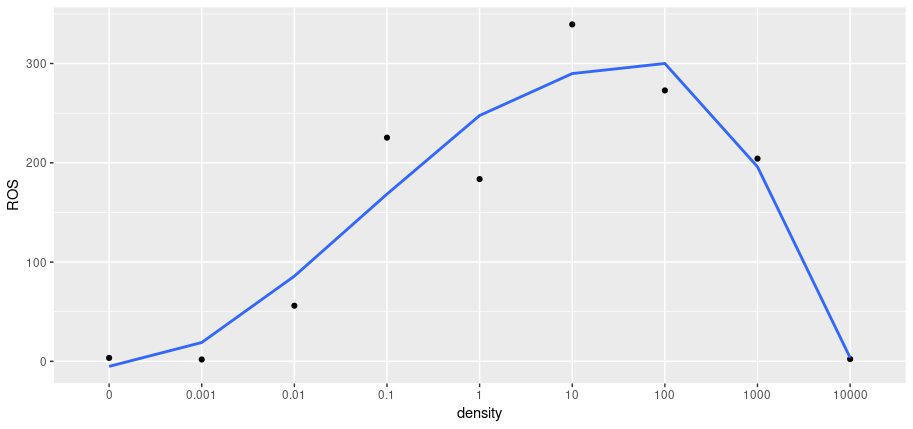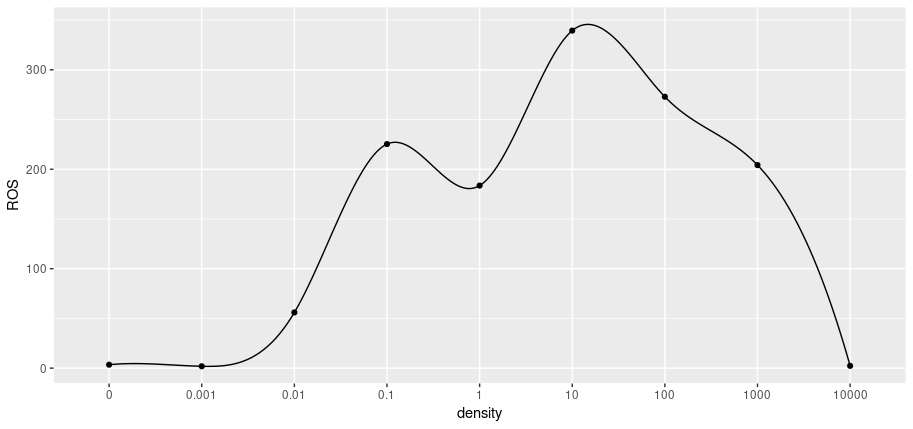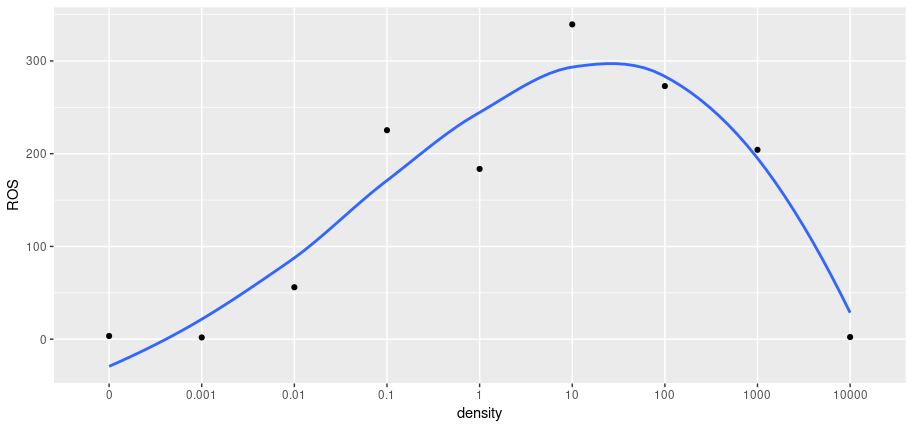# 利用 R 绘制拟合曲线

1. 利用 geomsmooth 进行曲线的拟合(method 选择 loess 或者添加 formula)；
2. 利用 spline 进行插值操作，然后用 geomline 进行连接。

 density ROS 0 3.43 0.001 1.86 0.01 56.00 0.1 225.31 1 183.56 10 339.40 100 272.89 1000 204.17 10000 2.29

## 单纯使用 goem_smooth

    library(ggplot2)
ros <- read.csv('tem.csv', colClasses = c('character', 'numeric'))
ggplot(ros, aes(x=density, y=ROS)) +
geom_point() +
geom_smooth(aes(x=density2, y=ROS), se = F, method = 'loess')## 单纯使用 spline

    ros <- transform.data.frame(ros, density2=1:9)
tem <- as.data.frame(spline(ros$density2, ros$ROS, n=10000))
ggplot(ros, aes(x=density, y=ROS)) +
geom_point() +
geom_line(data = tem, aes(x=x, y=y))## 同时使用前两种方法

    tem2 <- as.data.frame(spline(ros$density2, ros$ROS, n=100))
ggplot(ros, aes(x=density, y=ROS)) +
geom_point() +
geom_smooth(data = tem2, aes(x=x, y=y), se = F, method = 'loess')posted @ 2020-07-01 12:28  飞舞的冰龙  阅读(880)  评论(0编辑  收藏